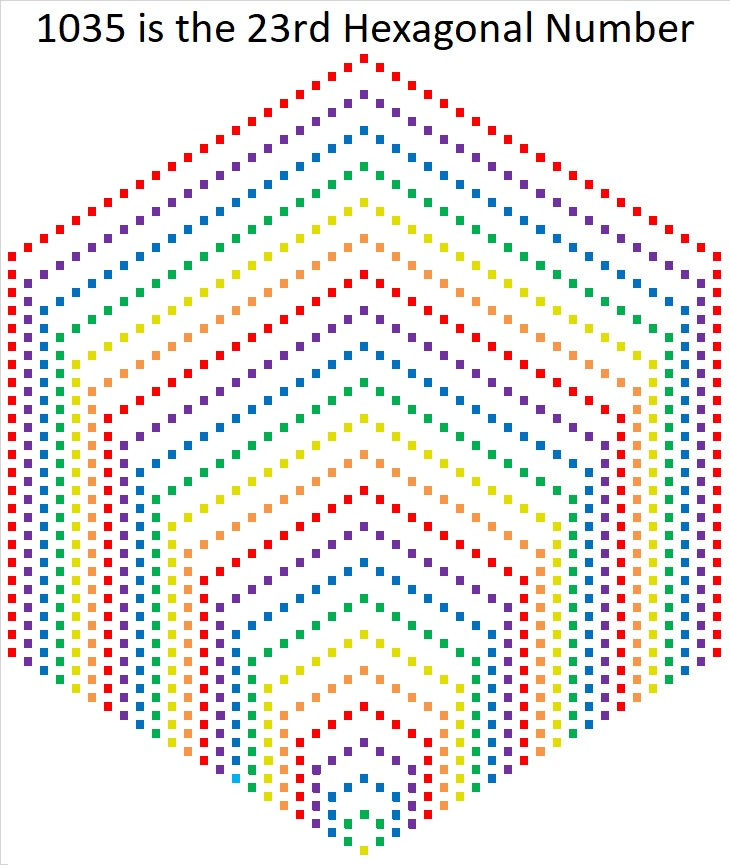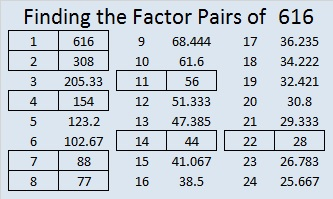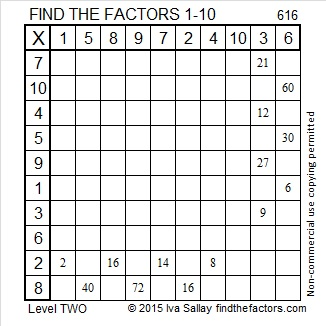# 1035 is the 23rd Hexagonal Number

1035 is the 23rd hexagonal number because of the way that it can be factored:
2(23²) – 23 = 1035,
(2(23) – 1)23 = 1035
or simply 45(23) = 1035.Starting at the bottom of the hexagonal we see 1 yellow square, 5 green squares, 9 blue squares, 13 purple squares, 17 red squares, and 21 orange squares.

1, 5, 9, 13, 17, 21, . . . is an arithmetic progression or arithmetic sequence. The common difference between the numbers is 4.

The nth hexagonal number is the sum of the first n numbers in that arithmetic progression.
The first few hexagonal numbers form an arithmetic series: 1, 6, 15, 28, 45, 66 and so forth.
1035 is the sum of the first 23 numbers in the progression so it is the 23rd term in the series and the 23rd hexagonal number.

All hexagonal numbers are also triangular numbers. 1035 is the 45th triangular number because 45(46)/2 = 1035.Starting in the lower left-hand corner of that triangle we see 1 yellow square, 2 green squares, 3 blue squares, 4 purple squares, 5 red squares, and 6 orange squares.

1, 2, 3, 4, 5, 6, . . .  is the simplest arithmetic progression there is. The common difference is 1.

The nth triangular number is the sum of the first n numbers in that arithmetic progression.

The first few triangular numbers form an arithmetic series: 1, 3,  6, 10, 15, 21, 28, 36, 45, 55, 66 and so forth.  (The blue triangular numbers are also hexagonal numbers.)
1035 is the sum of the first 45 numbers in the progression so it is the 45th term in the series and the 45th triangular number.

1035 is also the hypotenuse of one Pythagorean triple:
621-828-1035 which is (3-4-5) times 207

It is also a leg in several Pythagorean triples including
1035-1380-1725 which is (3-4-5) times 345

• 1035 is a composite number.
• Prime factorization: 1035 = 3 × 3 × 5 × 23, which can be written 1035 = 3² × 5 × 23
• The exponents in the prime factorization are 2, 1, and 1. Adding one to each and multiplying we get (2 + 1)(1 + 1)(1 + 1) = 3 × 2 × 2 = 23. Therefore 1035 has exactly 12 factors.
• Factors of 1035: 1, 3, 5, 9, 15, 23, 45, 69, 115, 207, 345, 1035
• Factor pairs: 1035 = 1 × 1035, 3 × 345, 5 × 207, 9 × 115, 15 × 69, or 23 × 45,
• Taking the factor pair with the largest square number factor, we get √1035 = (√9)(√115) = 3√115 ≈ 32.1714# 616 and Level 2

The Padovan sequence produces a lovely spiral of equilateral triangles similar to the spiral made from golden rectangles and the Fibonacci sequence.

The Padovan sequence begins with the following numbers: 1, 1, 1, 2, 2, 3, 4, 5, 7, 9, 12, 16, 21, 28, 37, 49, 65, 86, 114, 151, 200, 265, 351, 465, 616, 816, 1081 …

The first two numbers in the Fibonacci sequence are both 1’s. After that a number, n, in the Fibonacci sequence is found by adding together (n-2) and (n-1).

The first three numbers in the Padovan sequence are all 1’s. After that a number, n, in the Padovan sequence is found by adding together (n-3) and (n-2), and 616 is one of those numbers.Print the puzzles or type the solution on this excel file: 10 Factors 2015-09-14

—————————————————————————————————

• 616 is a composite number.
• Prime factorization: 616 = 2 x 2 x 2 x 7 x 11, which can be written 616 = (2^3) x 7 x 11
• The exponents in the prime factorization are 1, 3, and 1. Adding one to each and multiplying we get (3 + 1)(1 + 1)(1 + 1) = 4 x 2 x 2 = 16. Therefore 616 has exactly 16 factors.
• Factors of 616: 1, 2, 4, 7, 8, 11, 14, 22, 28, 44, 56, 77, 88, 154, 308, 616
• Factor pairs: 616 = 1 x 616, 2 x 308, 4 x 154, 7 x 88, 8 x 77, 11 x 56, 14 x 44, or 22 x 28
• Taking the factor pair with the largest square number factor, we get √616 = (√4)(√154) = 2√154 ≈ 24.819347.—————————————————————————————————